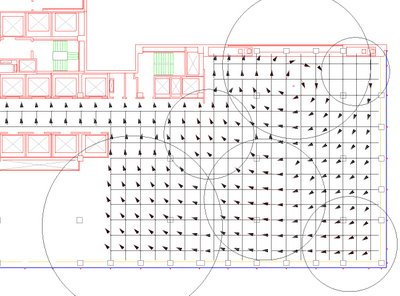## Monday, January 15, 2007

### 070115_rh4_VectorField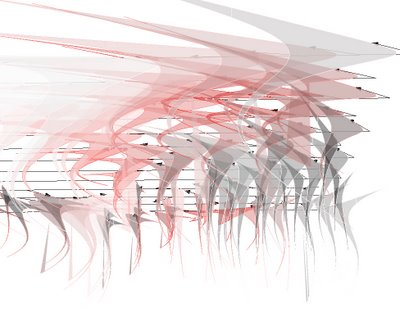"LE CHAMP" is a study for a small gallery for architecture in NYC; within the host of that SOM project with a very low budget and a tight scheddule, marc fornes & theverymany is investigating the notion of field and distributed design.

In order to challenge a program requiring a certain flexibility and still argue with the Modern notion of "open space", theverymany is following a trend defined in the 90's by people such as Greg Lynn who argues -in his book "Animate forms"- that boat hulls design is driven towards performances within very different types of sea and streams without actually using mechanical devices to change their morphologies...
though, since the argument is less valide as himself is challenging his students toward "Gigantic robots" and racing boats do integrate mechanical changes like the wings of some US military air fighters...
to be totally obsolete within the teswting field, those planes are actually looking at technology abble to mutate -material with memory- instead of shifting...

from "animate" rules, to shift in morphology, to mutate... back for the purpose of the exercice (lacking of US army budget and time frame developpment) to "animate" as a possible result of differnet forecast, the flow -within "LE CHAMP"- has been the most litterally expressed via a field vectors, actual device support for presentation panels which should be simply laser-cut onto a full spectrum of colored acrylic panels.

project protocol written in rhinoscript (rhino v4.0)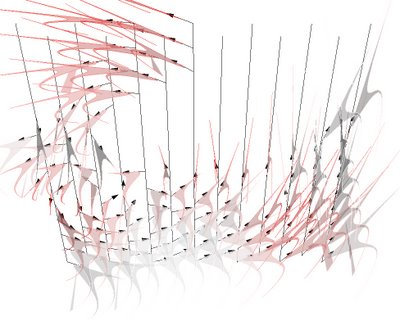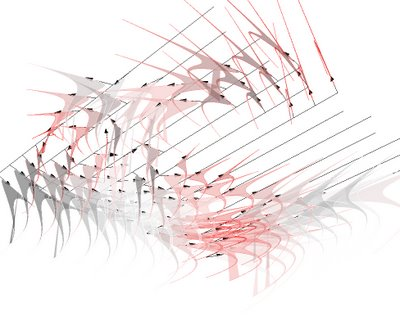VECTOR FIELD (Wikipedia.org)
In mathematics a VECTOR FIELD is a construction in vector calculus which associates a vector to every point in a Euclidean space.

Vector fields are often used in physics to model for example the speed and direction of a moving fluid throughout space, or the strength and direction of some force, such as the magnetic or gravitational force, as it changes from point to point.

In the rigorous mathematical treatment, (tangent) vector fields are defined on manifolds as sections of the manifold's tangent bundle.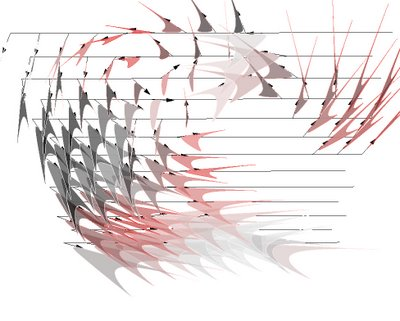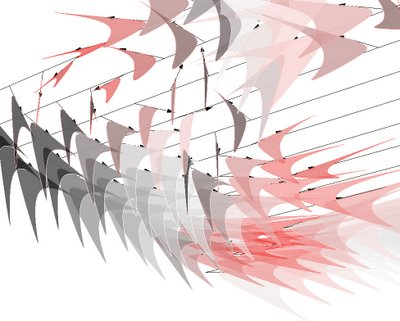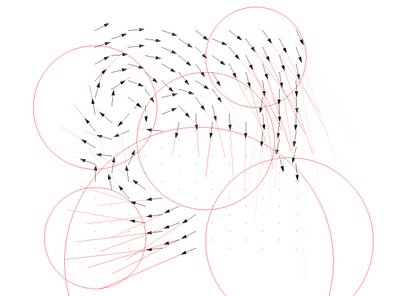FIELD LINE (Wikipedia.org)
A FIELD LINE is a locus that is defined by a vector field and a starting location within the field. A vector field defines a direction at all points in space; a field line may be constructed by tracing a path in the direction of the vector field.

Field lines are useful for visualizing vector fields, which consist of a separate individual vector for every location in space. If the vector field describes a velocity field, then the field lines follow stream lines in the flow. Perhaps the most familiar example of a vector field described by field lines is the magnetic field, which is often depicted using field lines emanating from a magnet.

A complete description of the geometry of all the field lines of a vector field is exactly equivalent to a complete description of the vector field itself.

Field lines can be used to trace familiar quantities from vector calculus: divergence may be seen as a net geometric divergence of field lines away from (or convergence toward) a small region, and the curl may be seen as a helical shape of field lines.

While field lines are a "mere" mathematical construction, in some circumstance they take on physical significance. In the context of plasma physics, electrons or ions that happen to be on the same field line interact strongly, while particles on different field lines in general do not interact.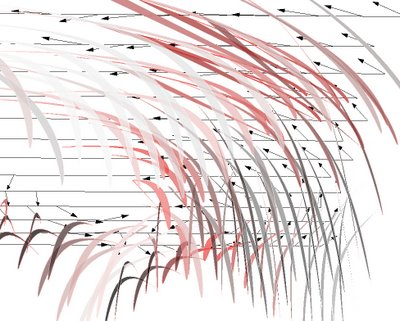VECTOR FIELD (mathworld.com)
A vector field is a map f:R^n|->R^n that assigns each x a vector function f(x). In French, a vector field is called "un champ." Several vector fields are illustrated above. A vector field is uniquely specified by giving its divergence and curl within a region and its normal component over the boundary, a result known as Helmholtz's theorem (Arfken 1985, p. 79).

Flows are generated by vector fields and vice versa. A vector field is a tangent bundle section of its tangent bundle.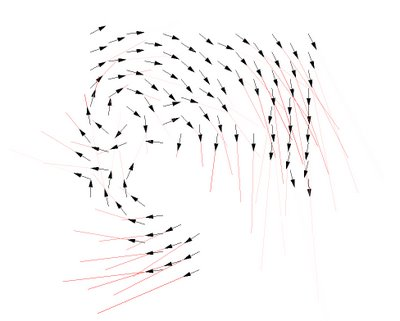RHINOSCRIPTING: "tips'n tricks: or where to start from for rhinoscript beginners"

Option Explicit
'code written by David Rutten (www.reconstructivism.net) within RhinoScript101_e
'This script will compute a bunch of cross-product vector based on a pointcloudVectorField()
Sub VectorField()
Dim strCloudID
strCloudID = Rhino.GetObject("Input pointcloud", 2, True, True)
If IsNull(strCloudID) Then Exit Sub
Dim arrPoints : arrPoints = Rhino.PointCloudPoints(strCloudID)
Dim ptBase : ptBase = Rhino.GetPoint("Vector field base point")
If IsNull(ptBase) Then Exit Sub
Dim i
For i = 0 To UBound(arrPoints)
Dim vecBase vecBase = Rhino.VectorCreate(arrPoints(i), ptBase)
Dim vecDir : vecDir = Rhino.VectorCrossProduct(vecBase, Array(0,0,1))
If Not IsNull(vecDir) Then vecDir = Rhino.VectorUnitize(vecDir) vecDir = Rhino.VectorScale(vecDir, 2.0)
End If
Next
End Sub

On Error Resume Next
If IsNull(ptBase) Or Not IsArray(ptBase) Then
ptBase = Array(0,0,0)
End If
Dim ptTip